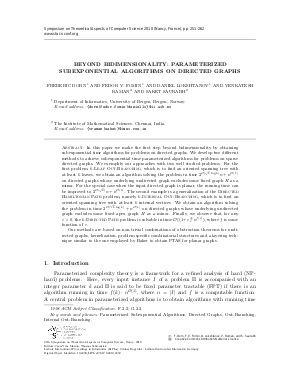Document# Beyond Bidimensionality: Parameterized Subexponential Algorithms on Directed Graphs

### Authors Frederic Dorn, Fedor V. Fomin, Daniel Lokshtanov, Venkatesh Raman, Saket Saurabh## File

LIPIcs.STACS.2010.2459.pdf
• Filesize: 316 kB
• 12 pages

## Cite As

Frederic Dorn, Fedor V. Fomin, Daniel Lokshtanov, Venkatesh Raman, and Saket Saurabh. Beyond Bidimensionality: Parameterized Subexponential Algorithms on Directed Graphs. In 27th International Symposium on Theoretical Aspects of Computer Science. Leibniz International Proceedings in Informatics (LIPIcs), Volume 5, pp. 251-262, Schloss Dagstuhl - Leibniz-Zentrum für Informatik (2010)
https://doi.org/10.4230/LIPIcs.STACS.2010.2459

## Abstract

In this paper we make the first step beyond bidimensionality by obtaining subexponential time algorithms for problems on directed graphs. We develop two different methods to achieve subexponential time parameterized algorithms for problems on sparse directed graphs. We exemplify our approaches with two well studied problems. For the first problem, $k$-Leaf Out-Branching, which is to find an oriented spanning tree with at least $k$ leaves, we obtain an algorithm solving the problem in time $2^{\cO(\sqrt{k} \log k)} n+ n^{\cO(1)}$ on directed graphs whose underlying undirected graph excludes some fixed graph $H$ as a minor. For the special case when the input directed graph is planar, the running time can be improved to $2^{\cO(\sqrt{k} )}n + n^{\cO(1)}$. The second example is a generalization of the {\sc Directed Hamiltonian Path} problem, namely $k$-Internal Out-Branching, which is to find an oriented spanning tree with at least $k$ internal vertices. We obtain an algorithm solving the problem in time $2^{\cO(\sqrt{k} \log k)} + n^{\cO(1)}$ on directed graphs whose underlying undirected graph excludes some fixed apex graph $H$ as a minor. Finally, we observe that for any $\ve>0$, the $k$-Directed Path problem is solvable in time $\cO((1+\ve)^k n^{f(\ve)})$, where $f$ is some function of $\ve$. Our methods are based on non-trivial combinations of obstruction theorems for undirected graphs, kernelization, problem specific combinatorial structures and a layering technique similar to the one employed by Baker to obtain PTAS for planar graphs.
##### Keywords
• Parameterized Subexponential Algorithms
• Directed Graphs
• Out-Branching
• Internal Out-Branching

## Metrics

• Access Statistics
• Total Accesses (updated on a weekly basis)
0